#### You may also like### Consecutive Numbers

An investigation involving adding and subtracting sets of consecutive numbers. Lots to find out, lots to explore.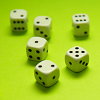### Roll These Dice

Roll two red dice and a green dice. Add the two numbers on the red dice and take away the number on the green. What are all the different possible answers?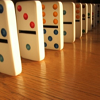### Domino Square

Use the 'double-3 down' dominoes to make a square so that each side has eight dots.

# Super Shapes

##### Age 7 to 11 ShortChallenge Level

Super Shapes printable sheet

Each of the following shapes has a value: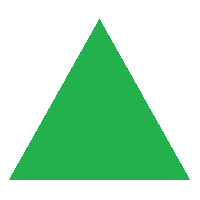$= 7$$= 17$

Can you discover the values of the other shapes using the information below?

 (a)+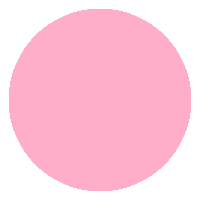+$= 25$ (b)+++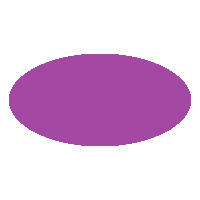$= 51$ (c)++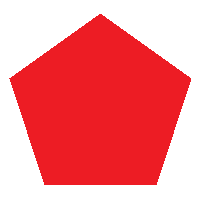+++$=136$ (d)++$=48$ (e)++++++$=100$